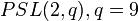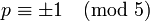# Alternating group:A6

View a complete list of particular groups (this is a very huge list!)[SHOW MORE]

## Definition

The alternating group$A_6$ is defined in the following equivalent ways:

## Arithmetic functions

Want to compare and contrast arithmetic function values with other groups of the same order? Check out groups of order 360#Arithmetic functions
Function Value Similar groups Explanation
order (number of elements, equivalently, cardinality or size of underlying set) 360 groups with same order As$A_n, n= 6$:$n!/2 = 6!/2 = 360$
As$PSL(2,q), q = 9$,$q^3 - q = 9^3 - 9 = 720$
exponent of a group 60 groups with same order and exponent of a group | groups with same exponent of a group Elements of order$3,4,5$.
derived length -- -- not a solvable group.
nilpotency class -- -- not a nilpotent group.
Frattini length 1 groups with same order and Frattini length | groups with same Frattini length Frattini-free group: intersection of maximal subgroups is trivial.
minimum size of generating set 2 groups with same order and minimum size of generating set | groups with same minimum size of generating set
subgroup rank of a group 2 groups with same order and subgroup rank of a group | groups with same subgroup rank of a group --
max-length of a group 5 groups with same order and max-length of a group | groups with same max-length of a group --

## Group properties

Property Satisfied Explanation Comment
Abelian group No$(1,2,3)$,$(1,2,3,4,5)$ don't commute$A_n$ is non-abelian,$n \ge 4$.
Nilpotent group No Centerless: The center is trivial$A_n$ is non-nilpotent,$n \ge 4$.
Metacyclic group No Simple and non-abelian$A_n$ is not metacyclic,$n \ge 4$.
Supersolvable group No Simple and non-abelian$A_n$ is not supersolvable,$n \ge 4$.
Solvable group No$A_n$ is not solvable,$n \ge 5$.
Simple non-abelian group Yes alternating groups are simple, projective special linear group is simple
T-group Yes Simple and non-abelian
Ambivalent group Yes Classification of ambivalent alternating groups
Rational-representation group No
Rational group No
Complete group No Conjugation by odd permutations in$S_6$ gives outer automorphisms.

## Elements

Further information: element structure of alternating group:A6

## Subgroups

Further information: subgroup structure of alternating group:A6

## Supergroups

Further information: supergroups of alternating group:A6

### Subgroups: making some or all the outer automorphisms inner

All the groups listed here are almost simple groups, because alternating group:A6 is a simple non-abelian group.

The outer automorphism group of alternating group:A6 is a Klein four-group. In particular, it has order 4. By the fourth isomorphism theorem, subgroups of the automorphism group containing the inner automorphism group correspond to subgroups of the outer automorphism group, which is the quotient group of the automorphism group by the inner automorphism group.

Since alternating group:A6 is a centerless group, it is identified naturally with its inner automorphism group, so each of the subgroups of the automorphism group containing the inner automorphism group is also a group containing$A_6$ as a self-centralizing normal subgroup. The whole automorphism group contains$A_6$ as a NSCFN-subgroup.

Below is the complete list of these groups.:

Group containing$A_6 = \operatorname{Inn}(A_6)$ and contained in$\operatorname{Aut}(A_6)$ (this is an almost simple group) Corresponding subgroup of$\operatorname{Out}(A_6)$ viewed as Klein four-group Order of group Order of corresponding subgroup of$\operatorname{Out}(A_6)$ = index of$A_6$ in group = order of group/360 Second part of GAP ID of big group (GAP ID is (order,2nd part))
alternating group:A6 trivial subgroup 360 1 118
symmetric group:S6 one of the three copies of Z2 in V4 720 2 763
projective general linear group:PGL(2,9) one of the three copies of Z2 in V4 720 2 764
Mathieu group:M10 one of the three copies of Z2 in V4 720 2 765
automorphism group of alternating group:A6 whole group 1440 4 5841

### Quotients: Stem extensions and Schur covering groups

All the groups listed here are quasisimple groups, because alternating group:A6 is a simple non-abelian group.

The Schur multiplier of alternating group:A6 is cyclic group:Z6, i.e., the group$\mathbb{Z}/6\mathbb{Z}$. For each of the possible quotient groups of$\mathbb{Z}/6\mathbb{Z}$, there is a unique stem extension with that as base normal subgroup and alternating group:A6 as quotient. The stem extension for the whole Schur multiplier is the unique Schur covering group, also called the universal central extension.

Note that uniqueness follows from$A_6$ being a perfect group.

The list is below:

Group at base of stem extension Order Corresponding stem extension group (this is a quasisimple group) Order Second part of GAP ID (GAP ID is (order,second part))
trivial group 1 alternating group:A6 360 118
cyclic group:Z2 2 special linear group:SL(2,9), also denoted$2 \cdot A_6$ to indicate that it is a double cover of alternating group 720 409
cyclic group:Z3 3 triple cover of alternating group:A6 1080 260
cyclic group:Z6 6 Schur cover of alternating group:A6 2160 (ID not available for this order)

## Linear representation theory

Further information: linear representation theory of alternating group:A6

### Summary

Item Value
Degrees of irreducible representations over a splitting field (such as$\mathbb{C}$ or$\overline{\mathbb{Q}}$) 1,5,5,8,8,9,10
grouped form: 1 (1 time), 5 (2 times), 8 (2 times), 9 (1 time), 10 (1 time)
maximum: 10, lcm: 360, number: 7, sum of squares: 360
Ring generated by character values$\mathbb{Z}[2\cos(2\pi/5)] = \mathbb{Z}[(1 + \sqrt{5})/2]$
Minimal splitting field, i.e., field of realization of all irreducible representations (characteristic zero)$\mathbb{Q}(\sqrt{5})$
Quadratic extension of$\mathbb{Q}$
Same as field generated by character values
Orbits of irreducible representations under action of automorphism group orbits of size 1 for representations of degree 1,9,10; orbits of size two for degree 5 and degree 8 representations (the degree 8 representations are interchanged under conjugation by an odd permutation; the degree 5 representations are interchaged by an automorphism that is outer for$S_6$ as well)
Orbits of irreducible representations under action of Galois group orbits of size 1 for representations of degree 1,5,5,9,10; orbit of size two for degree 8 representations (automorphism$\sqrt{5} \mapsto -\sqrt{5}$)
Minimal splitting field in prime characteristic$p \ne 2,3,5$ Case$p \equiv \pm 1 \pmod 5$: prime field$\mathbb{F}_p$
Case$p \equiv \pm 2 \pmod 5$: quadratic extension$\mathbb{F}_{p^2}$ of$\mathbb{F}_p$
Smallest size splitting field field:F11
Degrees of irreducible representations over the rational numbers 1,5,5,9,10,16

## GAP implementation

### Group ID

This finite group has order 360 and has ID 118 among the groups of order 360 in GAP's SmallGroup library. For context, there are 162 groups of order 360. It can thus be defined using GAP's SmallGroup function as:

SmallGroup(360,118)

For instance, we can use the following assignment in GAP to create the group and name it$G$:

gap> G := SmallGroup(360,118);

Conversely, to check whether a given group$G$ is in fact the group we want, we can use GAP's IdGroup function:

IdGroup(G) = [360,118]

or just do:

IdGroup(G)

to have GAP output the group ID, that we can then compare to what we want.

### Other descriptions

Description Functions used
AlternatingGroup(6) AlternatingGroup
PSL(2,9) PSL